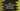# Java Arrays hashCode() method explanation with examples## Java Arrays hashCode() method explanation with examples:

Java Arrays hashCode method is used to return a hash code for an array. It gives the hash code value based on its content.

This method is defined in the java.util package in the Arrays class. This is a static method and it has variants for different types of arrays. The java.util.Arrays class provides different types of array manipulating methods.

In this post, we will learn how to use this method to find the hash code of different types of arrays with examples.

### Definition of hashCode:

hashCode method is defined for boolean arrays, byte arrays, char arrays, double arrays, float arrays, int arrays, long arrays, Object arrays and short arrays. These

``````public static int hashCode(boolean[] arr)

public static int hashCode(byte[] arr)

public static int hashCode(char[] arr)

public static int hashCode(double[] arr)

public static int hashCode(float[] arr)

public static int hashCode(long[] arr)

public static int hashCode(int[] arr)

public static int hashCode(Object[] arr)

public static int hashCode(short[] arr)``````

As you can see here,

• This is a static method. We don’t have to create any object for the Arrays class to use this method. We can use it directly.
• arr is the array we are finding the hash code.
• This method returns a hash code based on the contents of the array.
• If the Arrays.equals method returns true for two different arrays, their hash code will be equal, i.e. the hashCode method will return the same value for both.
• If we insert these items in the same order to a List, and call the hashCode method, it will return the same value.

### Example of hashCode with an integer array:

Let’s learn how hashCode method works with an integer array.

``````import java.util.Arrays;

class Main {
public static void main(String[] args) {
int[] firstArray = {1, 2, 3, 4, 5, 6, 7};
int[] secondArray = {1, 2, 3, 4, 5, 6, 8};
int[] thirdArray = {1, 2, 3, 4, 5, 6, 7};

System.out.println("Arrays.hashCode of firstArray: " + Arrays.hashCode(firstArray));
System.out.println("Arrays.hashCode of secondArray: " + Arrays.hashCode(secondArray));
System.out.println("Arrays.hashCode of thirdArray: " + Arrays.hashCode(thirdArray));
}
}``````

If you run this program, it will print:

``````Arrays.hashCode of firstArray: -1604500253
Arrays.hashCode of secondArray: -1604500252
Arrays.hashCode of thirdArray: -1604500253``````
• firstArray and thirdArray are equal, but secondArray is different.
• You can see that Arrays.hashCode method returns the same value for both firstArray and thirdArray but it returns a different value for secondArray.

### Example of hashCode with an object array:

Let’s use Arrays.hashCode with an array of objects. We will create a class and objects for this class with an array. Let me show you the program:

``````import java.util.Arrays;

class Student {
String name;
int age;

public Student(String name, int age) {
this.name = name;
this.age = age;
}
}

class Main {
public static void main(String[] args) {
Student firstStudent = new Student("Alex", 10);
Student secondStudent = new Student("Bob", 20);

Student[] firstArray = {firstStudent, secondStudent};
Student[] secondArray = {firstStudent, secondStudent};
Student[] thirdArray = {new Student("Alex", 10), new Student("Bob", 20)};

System.out.println("Arrays.hashCode of firstArray: " + Arrays.hashCode(firstArray));
System.out.println("Arrays.hashCode of secondArray: " + Arrays.hashCode(secondArray));
System.out.println("Arrays.hashCode of thirdArray: " + Arrays.hashCode(thirdArray));
}
}``````

Here,

• Student is the class that can hold the name and age of a student.
• We created two objects firstStudent and secondStudent with different name and age values.
• We created two arrays firstArray and secondArray with these objects. Also another array thirdArray is created with two Student objects. These objects have same name and age values as the firstStudent and secondStudent objects.

If you run this program, it will print:

``````Arrays.hashCode of firstArray: -640084409
Arrays.hashCode of secondArray: -640084409
Arrays.hashCode of thirdArray: -95519366``````

As you can see here, Arrays.hashCode returned the same values for the firstArray and secondArray, but it returned a different value for thirdArray. Even though thirdArray contains objects with similar content, the return of Arrays.hashCode is different because they are not referring to the exact object.

### Example with null arrays:

Arrays.hashCode returns 0 if we pass a null array.

``````import java.util.Arrays;

class Main {
public static void main(String[] args) {
int[] arr = null;
System.out.println("Arrays.hashCode of null: " + Arrays.hashCode(arr));
}
}``````

It will print 0.